## kernelkmeans.m

From A First Course in Machine Learning, Chapter 6. Simon Rogers, 01/11/11 [simon.rogers@glasgow.ac.uk] Kernel K-means

```clear all;close all;
```

```load ../data/kmeansnonlindata
```

## Plot the data

```figure(1);hold off
plot(X(:,1),X(:,2),'ko');
```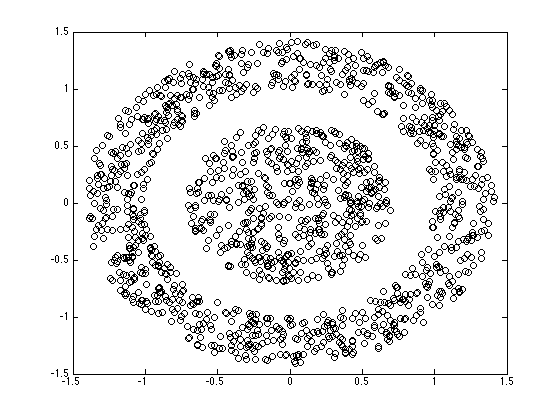## Compute the kernel

```N = size(X,1);
Ke = zeros(N);
gam = 1;
for n = 1:N
for n2 = 1:N
Ke(n,n2) = exp(-gam*sum((X(n,:)-X(n2,:)).^2));
end
end
```

## Run Kernel K-means

```converged = 0;
% Assign all objects into one cluster except one
% Kernel K-means is *very* sensitive to initial conditions.  Try altering
% this initialisation to see the effect.
K = 2;
Z = repmat([1 0],N,1);
s = sum(X.^2,2);
pos = find(s==min(s));
Z(pos,:) = [0 1];
di = zeros(N,K);
cols = {'r','b'};
```

## Plot the assignments

```figure(1);hold off
for k = 1:K
pos = find(Z(:,k));
plot(X(pos,1),X(pos,2),'ko','markerfacecolor',cols{k});
hold on
end
``````while ~converged
```
```    Nk = sum(Z,1);
for k = 1:K
% Compute kernelised distance
di(:,k) = diag(Ke) - (2/(Nk(k)))*sum(repmat(Z(:,k)',N,1).*Ke,2) + ...
Nk(k)^(-2)*sum(sum((Z(:,k)*Z(:,k)').*Ke));
end
oldZ = Z;
Z = (di == repmat(min(di,[],2),1,K));
Z = 1.0*Z;
if sum(sum(oldZ~=Z))==0
converged = 1;
end
```

## Plot the assignments

```    figure(1);hold off
for k = 1:K
pos = find(Z(:,k));
plot(X(pos,1),X(pos,2),'ko','markerfacecolor',cols{k});
hold on
end
```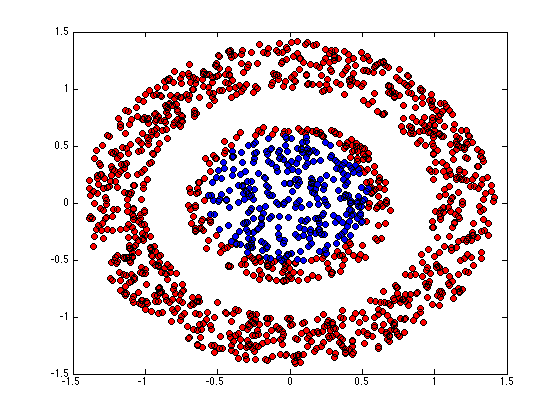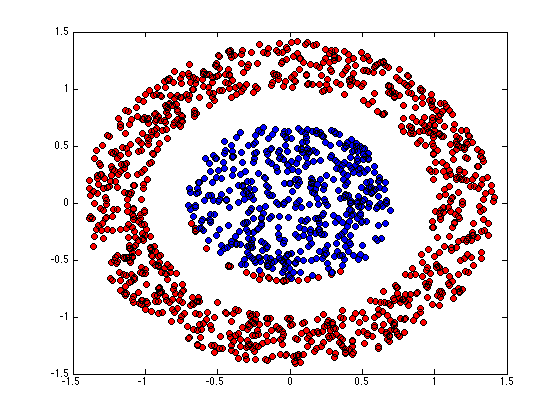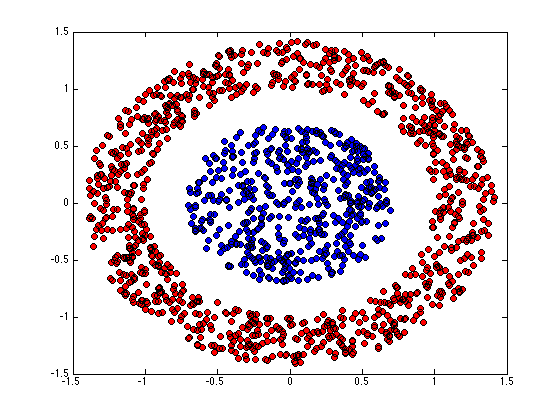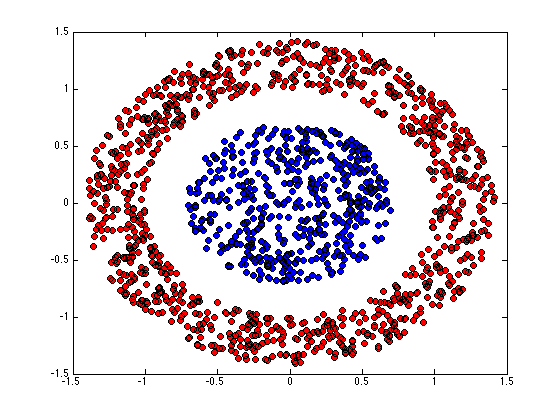```end
```